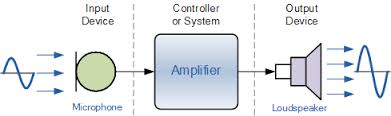# Strain gauge transducer types and working ,priciple

## Strain gauge transducer types and working

Strain gauge is a type of transducer which is used to measure  Strain ( Linear or angular force) force.

###working Principle of Strain gauge transducer

We know that metal resistance is directly proportional to its length, Hence whenever  pull any metal ( Applied tensile force) or compress them ( Applied compress force) so change metal resistance from different reason.

1.  Change in length
2. Change in diameter
3. Change in resistivity

By using a electric circuit we measure the changes of resistance and by use this also measure applied force

According figer when applied tensile force on metal his diameter varies from “D” to  “d ”  and their length also varies  “L” to “l” so resistance are also varies from “R” to “r”so gauge factor

GF =  r/R divide by l/L

GF = 1 to 2

### Type of strain gauge transducer

1. Unbounded type strain gauge
2. Bonded type strain gauge
3.Bonded metal foil strain gauge
4. Semiconductor Strain gauge

### 1. Wheatstone bridge strain gauge transducer

Unbound type strain gauge made from different material
1. Copper-Nickel
2. Chrome-Nickel
3. Nickel-Iron

In this material main quality available which is elasticity which means when  force applied on metal length and diameter will change but remove this force its take his original position. It has four arms which connect like wheat stone bridge.
Wheatstone bridge condition
P/Q = R/S
where p q r s is resistance
Voltage E = 0  ( in balance condition)
when force applied on diaphragm unbalance create and resistance change so out put terminal get o/p volatile . which is strain force, ( this voltage is directly proportional to applied force ) . so voltmeter shows reading of applied force

### 2. Bonded type strain gauge

In bonded type strain gauge we use paper , bake light , teflon on grid element . it use like a base to protect from wire and also provide mechanical protection.

### 3. Bonded metal foil strain gauge

It is also type of Bonded type strain gauge  but in this type strain gauge polymide  or glass used as a grid base.

### 4. Semiconductor strain gauge

In semiconductor strain gauge silicon , germanium and other material used when applied force  more resistivity changes become  which used more force me can measure.

#### Advantage of semiconductor strain gauge

1. higher gauge factor

2. high sensitivity

3. Long life

4. Small size

5. Low cost

6. higher heat dissipation capacity